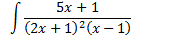Lec 6 - Integration by Rational Function by Partial FractionSec 7.4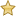Techniques of Integration

1. Substitution
1. Integration by parts
2. Trig Integrals
1. Trig substitutions
1. Partial functions

Recall,We will learn how to do this backwards so that the integrand can be rewritten as a sum of terms each of which we can be rewritten as a sum of terms each of which we can integrate.

Definition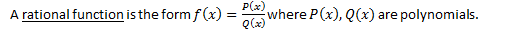We only apply partial fractions on proper rational functions.

If not proper then we long division to reduce itExample

Evaluate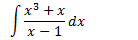We can use long division.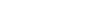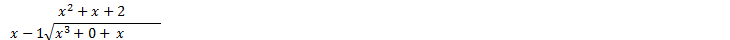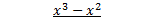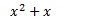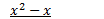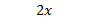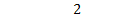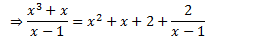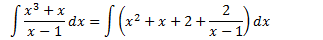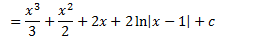Step 1: Use long division to get a proper fraction

Step2: Decompose our proper rational function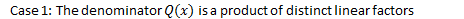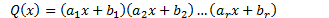In this case we always assume,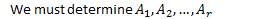The method I present is different from the textbook.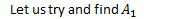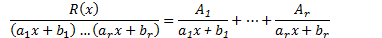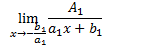On the LHS, we have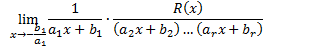Therefore,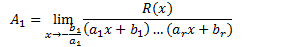Eg

Evaluate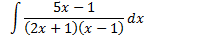Step 1: It is proper, no long division.

Step 2: We use partial fractions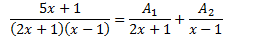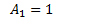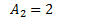Step 3: Evaluate